# Improvement and Application of Multi-layer LSTM Algorithm Based on Spatial-Temporal Correlation

Improvement and Application of Multi-layer LSTM Algorithm Based on Spatial-Temporal Correlation
Department of Mathematics and Computer Science, Hebei Normal University for Nationalities, Chengde 067000, China

Corresponding Author Email:
zhaoyanming008@163.com
Page:
49-58
|
DOI:
https://doi.org/10.18280/isi.250107
10 August 2019
|
Accepted:
17 December 2019
|
Published:
29 February 2020
| Citation

OPEN ACCESS

Abstract:

Current algorithms for the prediction of air pollutant particle concentration generally failed to effectively integrate with the time dependence and spatial correlation features of particle concentration. To this end, this paper studied the improvement and application of the multi-layer LSTM algorithm based on spatial-temporal correlation. First, the paper proposed the method for calculating the correlation coefficients of air pollutant particle concentration in global and local regions, and established the matrix for the corresponding correlation coefficients; then layer by layer, the K-1 layer LSTM algorithm was used to extract the time dependence eigen vector H of the particle concentration at N observation sites, and calculate the product (R) of the local correlation coefficient matrix and eigen vector H, so as to achieve the fusion of time dependence and spatial correlation features in local region; at last, at the K layer, the inner product of the global correlation coefficient matrix and R was calculated to extract the spatial correlation feature of particle concentration in global and local regions. On the global and local datasets, the proposed algorithm was compared with the LSTME algorithm, space-time deep learning (STDL) algorithm, time delay neural network (TDNN) algorithm, autoregressive moving average (ARMA) algorithm, support vector regression (SVR) algorithm and the traditional LSTM NN algorithm. The comparison results showed that, in terms of air particle concentration prediction, the proposed algorithm outperformed the other algorithms, proving that the multilayer neural network based on spatial-temporal correlation can effectively improve the prediction performance of the LSTM algorithm.

Keywords:

long-short term memory (LSTM) network, air pollutant concentration prediction, recurrent neural network (RNN), spatial-temporal correlation, PM2.5 concentration

1. Introduction

PM2.5 particles pose a serious threat to human health. In 2009, Krewski et al.  pointed out that there was an obvious correlation between sudden human death and the duration of exposure to PM2.5. In 2013, Zheng et al.  proposed that the real-time prediction of air pollutant concentration is of great significance for preventing diseases caused by the pollutant. In 2017 and 2018, Di et al.  and Hung et al.  respectively pointed out that the smaller the particle volume of the pollutant, the stronger the water solubility, the stronger the penetrability of the pollutant particles into the respiratory system, the higher the adsorption rate, and the greater the impact on human health. Therefore, the prediction of PM2.5 has become a current research hotspot.

Air pollutant concentration prediction algorithms mainly include two types: process model algorithms and statistical algorithms. With meteorological theories as priori knowledges, the process model algorithms simulate the generation, discharge, diffusion, conversion and removal of pollutants according to the atmospheric physicochemical reaction processes. Key process model algorithms include: spatial-temporal evolution feature simulation algorithm of physical and chemical reaction of air pollutants based on scale and direction , community multiscale air quality model (CMAQ) algorithm , embedded air quality prediction algorithm , and WRF-Chem model-based mesoscale air quality algorithm . However, Vautard et al.  and Stern et al.  pointed out that the process model algorithms could achieve better prediction results in air quality prediction, but they are limited by conditions such as complex prior knowledge, infinite data sets, and multidimensional restraints, therefore, such algorithms are often of poor universality.

To solve the problem of algorithm universality, the statistical algorithms had been proposed. Statistical algorithms construct air quality prediction models based on statistical theories, and the main methods include: autoregressive moving average (ARMA) method , multiple linear regression (MLR) , support vector regression (SVR)  and other regression methods, artificial neural network (ANN)  and its hybrid algorithms , the experiment of algorithm  showed that the nonlinear mapping, adaptive and robust features of ANN determined its good performance in time series prediction and the algorithm could be widely used. Typical ANN algorithms include: multilayer perceptron  (MLP), BP neural network , RBF neural network , fuzzy-decision neural network (FDNN) , general regression neural network  (GRNN), recurrent neural network  (RNN), time-delay neural network (TDNN) , and Elman neural networks , etc.

However, the problems of gradient vanishing or gradient exploding had restricted the long-term time-dependence feature of RNN in learning time series. To this end, in 1997, Hochreiter and Schmidhube  developed a LSTM (long-term and short-term memory) neural network (NN). Unlike traditional RNNs, the LSTM NN solved the gradient problems and realized long-term time dependence learning of time series. LSTM NN has been applied to the prediction of the evolution process of air pollutant particle concentration and achieved certain progress. Common LSTM algorithms include: ensemble-LSTM algorithm , CNN-LSTM algorithm , LSTM-FC algorithm ; the LSTM algorithm based on the features of air pollutant particle concentration: GC-LSTM algorithm , spatiotemporal convolutional LSTM algorithm ; the LSTM algorithm based on deep learning (DL-LSTM) , multi-output DL-LSTM algorithm ; and Deep CNN-LSTM algorithm .

In summary, LSTM algorithms have achieved good research results in the simulation of the evolution process of air pollutant particle concentration and the prediction of the concentration value, but they still have the following shortcomings: (1) The algorithms are mainly applied to the classification of atmospheric pollutants, rather than the evolution simulation and concentration prediction; (2) The algorithms have not explored the spatial correlation feature of PM2.5 in depth; (3) The algorithms have not effectively integrated the time dependence feature with the spatial correlation feature of pollutant particle concentration.

Therefore, this paper aims to study the simulation of the evolution process of air pollutant particle concentration and the prediction of the concentration value by integrating the time dependence and spatial correlation features extracted by the LSTM algorithm, and constructs the atmospheric evolution algorithm to predict the concentration of air pollutant particles. The main innovations of this paper include: (1) The paper defined the spatial correlation of PM2.5 particle concentration and its calculation method; (2) It used the multilayer LSTM network to learn the long-term time dependence feature of PM2.5 particle concentration; (3) According to the information of the spatial correlation feature of PM2.5 particle concentration, the paper proposed a neighbor correlation matrix generation algorithm and constructed the neighbor correlation matrix; (4) Integrated with the spatial-temporal correlation features of PM2.5 particle concentration, this paper constructed the TSM-LSTM algorithm (temporal-spatial multi-scale LSTM) for the prediction of air concentration and applied it to the accurate prediction of PM2.5 concentration.

2. LSTM Algorithm Based on Spatial-Temporal Correlation

The evolution of PM2.5 particle concentration is a stochastic process with both time dependence and spatial correlation features, and is restricted by many factors. Therefore, constructing a LSTM algorithm integrating both features is conductive to better simulating the evolution process of PM2.5 particle concentration and predicting the value of the concentration.

2.1 PM2.5 particle concentration evolution process

The evolution of PM2.5 particle concentration is affected by internal and external factors. Internal factors refer to the physical and chemical factors that produce PM2.5 particles and have the characteristic of slow-varying. In this paper, external factors refer to outside factors that would cause the evolution of PM2.5 particle concentration, including the time factor and the space factor. The time factor refers to the time dependence of the observation data at a same observation site. The space factor refers to the effect of the particle concentration of the neighbor observation site on the particle concentration of the current observation site.

Therefore, the evolution of PM2.5 particle concentration is a random process with temporal and spatial correlation features.

2.2 PM2.5 particle concentration has spatial correlation feature

Feng et al.  and Qi et al.  pointed out that the factors of the spatial correlation of PM2.5 particle concentration include: geographical location, regional mountains and wind, but they hadn’t taken account the factor of the vegetation between the observation sites, especially the effect of the mountain vegetation on the PM2.5 particle concentration. Therefore, the response map of the spatial correlation factors of PM2.5 particle concentration is shown in Figure 1, and the spatial correlation factor τ is expressed as follows:

## 1.pngFigure 1. Spatial correlation factors of PM2.5 particle concentration

The spatial correlation factor (also known as the global spatial correlation factor) τ is:

$\tau(\mathrm{i}, \mathrm{j})=\frac{\text{Wind_cofficent}\times \text{Mountain_cofficent}\times \text {Vegetation_cofficent}}{\mathrm{D}(\mathrm{i}, \mathrm{j})}$    (1)

where, Mountain_cofficent represents the mountain influence coefficient between the two adjacent points pj and pi, it can be expressed as Mountain_cofficent=M_length×M_width×M_high×cosφ, wherein the M_length, M_width, and M_high respectively represent the length, width, and height of the mountain, and angle f is the included angle between the trend of the mountain and the connection line Pij; Wind_cofficent represents the wind influence coefficient between points pj and pi during this period of time, it can be expressed as Wind_cofficent=W_wind×cosθ, wherein $W_{\text {wind}}$ represents the average wind strength between pj and pi during this period of time, and θ is the angle between the direction of the wind and the connection line of pj and pi; Vegetation_cofficent represents the vegetation influence coefficient between pj and pi, it reflects the effect of vegetation on the PM2.5 particle concentration at the observation site pj, Vegetation_cofficent=NDVI(i,j), and this coefficient is related to the flourishing degree of vegetation between pj and pi, this paper uses the NDVI coefficient to represent it, which can well reflect the flourishing degree of vegetation, and it is less affected by other conditions. D(j,i) represents the distance between observation points pj and pi, and it can be expressed by the Euclidean distance of the latitude and longitude between the two points.

Formula (1) shows the effect of factors such as geographical location, regional mountains and wind on the evolution of PM2.5 particle concentration at adjacent observation sites, therefore, the spatial correlation matrix of air pollutant particle concentration was constructed as follows:

## 2t.png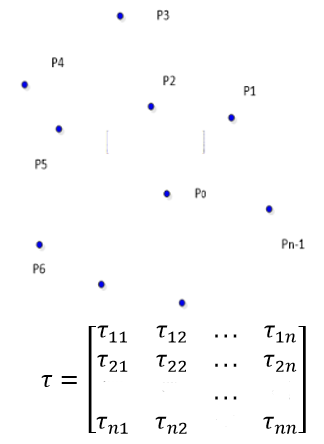τ(i,j) represents the spatial correlation coefficient of two neighbor air monitoring sites i and j, τ is the spatial correlation matrix between the sites within the region, it represents the coefficients of the influence of the air quality of each neighbor monitor site on the observation site.

As the sizes of the research area of research objects pi and pj were different, the effects of spatial correlation factors on the correlation coefficient τ varied as well; when the geographical area of the research object was relatively large, according to the effects of each factor, this paper defined the correlation coefficient as the global spatial correlation coefficient; when the geographical area of the research object was relatively small, and only wind and distance factors acted as the main factors, this paper defined the correlation coefficient as the local spatial correlation coefficient τ; and the calculation method was to simplify Formula (1).

The calculation method for the local spatial correlation factor τ of the i-th observation site to the j-th neighbor observation site is:

$\operatorname{Local}_{-} \tau(i, j)=\frac{\text {Wind_} \text {cofficent}}{D(i, j)}$    (2)

where, i and j are the observation sites. D(i,j) represents the Euclidean distance between the two sites. Therefore, the spatial correlation matrix E1 was defined as:

## 2t1.png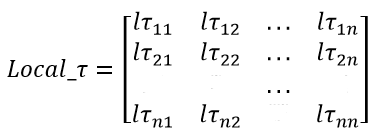The i-th row of E1 are the spatial correlation coefficients of all sites to the j-th site.

2.3 PM2.5 particle concentration has long-term time dependence feature

In the evolution of PM2.5 particle concentration, at an observation site, the particle concentration at a certain moment is affected by the particle concentration at the previous moment, and this effect has the feature of long-term time dependence, which can be expressed as:

$\psi(t, x, y)=\varphi(\psi(t-1, x, y), \psi(t-2, x, y),$

$\psi(t-3, x, y) \ldots, \psi(k, x, y))$    (3)

where, function f represents the long-term time-dependence of the time series. x,y represents the location of the observation site, denoted by latitude and longitude. The research in some literatures showed that the LSTM algorithms that can solve the gradient problems of RNN network could better analyze and learn the time dependence feature of PM2.5 particle concentration, and get better prediction and classification results.

2.4 Design and implementation of the LSTM algorithm based on spatial-temporal correlation

Based on the above analysis, this paper proposes the TSM-LSTM algorithm which first uses the LSTM algorithm to extract the time dependence feature of PM2.5 particle concentration and then integrates it with the spatial correlation feature calculated by Formula (1) to achieve the simulation of the evolution process of PM2.5 particle concentration and the effective prediction of PM2.5 particle concentration.

Design idea of the algorithm: first, use the multilayer LSTM NN to learn the time dependence feature of PM2.5 particle concentration time series of the observation sites; then use Formula (1) (2) to calculate the spatial correlation matrix between the observation sites, and use the inner product method to integrate the time dependence results with the spatial correlation matrix to learn the deep-level features of PM2.5 particle concentration, simulate the evolution process of PM2.5 particle concentration, and achieve the prediction of PM2.5 particle concentration.

The structure of the TSM-LSTM algorithm is as shown in Figure 2, which shows that the feature of the long-term time dependence of PM2.5 particle concentration was learned through the multilayer LSTM network. $\left\{x_{t_{1}}, x_{t_{2}}, x_{t_{3}}, \ldots . x_{t_{n}}\right\}$ represents the observation data series of the N sites with time lag. The time-lag parameter was set to be ∆. Subsequent experiments showed that parameter ∆ was related to the size of the research region, the larger the area of the research region, the longer the time lag.  represents the operation of the inner product of vectors.

In terms of the TSM-LSTM algorithm’s integration method of the time dependence and spatial correlation features, the integration of the time dependence and spatial correlation features of the PM2.5 particle concentration was achieved through two steps: integration of the information related to local space and the integration of the information related to global space. The integration method of local geographic information is as follows:

## 2.png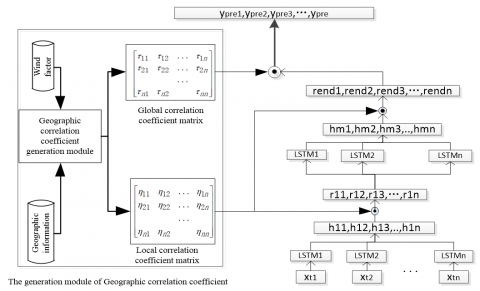Figure 2. Structure of the prediction algorithm of TSM-LSTM

For the i-th site, the integration method for the spatial and temporal correlations is:

$\rho_{i}\left(h_{l i}, \text { position }\right)=\operatorname{Local}_{-} \tau\left\{h_{l 1}, h_{l 2}, h_{l 3}, \ldots, h_{l n}\right\}$    (4)

hli represents the long-term time dependence information of the learning of the l-th layer of the LSTM network. $\rho_{i}\left(h_{l i}, \text { position }\right)$ represents the feature of the PM2.5 particle concentration after the l-th layer spatiotemporal information integration.

The implementation method for integrating the global geographic information is:

$\left(\begin{array}{lllll}y_{\text {pre1}} & y_{\text {pre2}} & y_{\text {pre3}} & \ldots & y_{\text {pren}}\end{array}\right)=\tau\left(\begin{array}{c}h_{\text {end} 1} \\ h_{\text {end} 2} \\ h_{\text {end} 3} \\ \ldots \\ h_{\text {endn}}\end{array}\right)$    (5)

where, yprei represents the predicted value of the PM2.5 concentration at the i-th observation site. hendi represents the predicted value of the PM2.5 concentration of the last layer of LSTM.

Through twice geographic information integration, the global and local PM2.5 particle concentration evolution processes were merged into one, which made the algorithm more universal and the prediction of PM2.5 particle concentration more accurate.

2.5 Evaluation methods for prediction algorithms

In this paper, the root means square error (RMSE), mean absolute error (MAE), and mean absolute percentage error (MAPE (%)) were taken as the evaluation criteria for the performance of the algorithms, which are expressed as follows:

$R M S E=\sqrt{\frac{1}{n} \sum_{i=1}^{N}\left(y_{i}-y_{i}^{*}\right)^{2}}$    (6)

$M A E=\frac{1}{n} \sum_{i=1}^{n}\left|y_{i}-y_{i}^{*}\right|$    (7)

$M A P E=\frac{1}{n} \sum_{i=1}^{n} \frac{\left|y_{i}-y_{i}^{*}\right|^{2}}{y_{i}^{*}}$    (8)

where, $y_{i}^{*}$ is the measured air pollutant concentration, $\mathcal{Y}_{i}$ is the predicted air pollutant concentration, and n is the number of the observation samples.

3. Experimental Datasets and Results

3.1 Research region of the algorithm and the validation of datasets

This paper uses global and local datasets to study the performance of the TSM-LSTM algorithm. The local dataset refers to the hourly PM2.5 concentration data set collected from 12 air quality monitoring sites in Beijing from January 1, 2015 to December 30, 2018. The local data set contained the main factors for the formation of PM2.5 in fast-developing megacities, and had good representativeness. The global dataset refers to the daily PM2.5 concentration data set collected from 12 air quality monitoring sites in Beijing city, Tianjin city and Hebei province from January 2013 to December 2018. The global data set contained the main factors for the formation of PM2.5 in developing countries, and had good representativeness. The global and local datasets were divided into a test set and a training set at a ratio of 20:80 (as shown in Figure 3 and Table 1).

## 3.png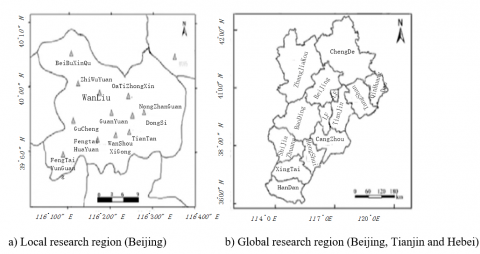Figure 3. Researching regions

## 4.png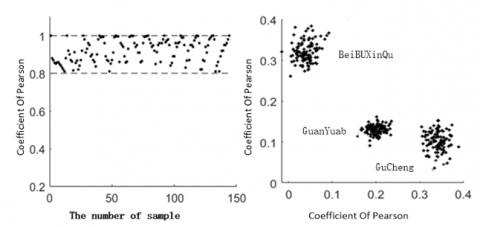Figure 4. Distribution map of Pearson correlation coefficients of PM2.5 concentration in local research region (∆=1h)

## 5.png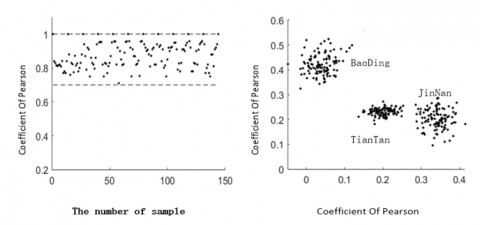Figure 5. Distribution map of Pearson correlation coefficients of PM2.5 concentration in global research region (∆=36h)

Table 1. Research regions

 Types of the region Research regions Local region North new district, Fengtai Yungang, National Agricultural Exhibition Center, Chengde, Langfang, Baoding, Shijiazhuang, Handan, Dongli, Jinnan, Development Zone and Wuqing District; Global region North new district, Botanical Garden, Wanliu, Olympic Sports Center, National Agricultural Exhibition Center, Dongsi, Guanyuan, Gucheng, Temple of Heaven, Wanshou West Palace, Fengtai Garden and Fengtai Yungang

3.2 Spatial correlation research based on Pearson theory

Taking the PM2.5 particle concentration data of 12 sites in Beijing as the observation objects, the Pearson correlation coefficient method was adopted to calculate the geographical correlation of the particle concentration at the 12 sites, and the Pearson correlation coefficient distribution map was plotted in Figure 4 and Figure 5.

The experimental results showed that the Pearson correlation coefficients of PM2.5 concentration at 12 air monitoring sites in Beijing urban area were all higher than 0.8, and the correlation coefficients of neighbor sites were higher than 0.91. Therefore, the PM2.5 concentrations of the 12 observation sites had a strong spatial correlation, and the correlation of the neighbor sites was higher than that of the distant sites.

The experimental results showed that the Pearson correlation coefficients of PM2.5 concentration at 12 air monitoring sites in Beijing-Tianjin-Hebei region were all higher than 7.3, and the correlation coefficients of neighbor sites were higher than 0.87. Therefore, the PM2.5 concentrations at the 12 observation sites had a strong spatial correlation, and the correlation of the neighbor sites was higher than that of the distant sites. Similar to the research results of 12 sites in Beijing, due to the value of time lag, the correlation coefficients of the global region were lower than those of the local region.

3.3 Long-term time dependence research based on autocorrelation

The autocorrelation coefficient method was used to calculate the autocorrelation coefficients of the PM2.5 concentrations at 12 air monitoring sites in the global and local regions, the curves of the autocorrelation coefficients were drawn as follows Figure 6.

## 6.png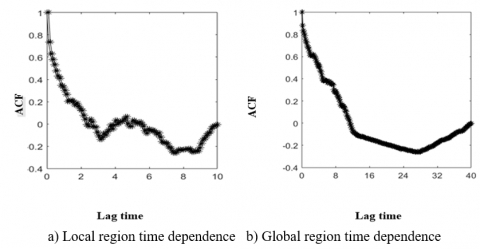Figure 6. Relationship between autocorrelation coefficient of PM2.5 particle concentration and time lag

The experimental results showed that, in the local and global regions, the particle concentrations between the observation sites had the long-term time dependence feature, and the time-lag relationship was quite obvious. Compared with the long-term time dependence of local region, the time-lag of the long-term time dependence of the global region was much longer.

3.4 The effect of time lag

The above experiments showed that the evolution process of PM2.5 particle concentration was affected by the time lag. Therefore, three criteria were set to evaluate the time-lag of different regions, and the evaluation results are shown in Table 2.

The experimental results showed that the time-lag had a significant effect on the performance of the algorithm. The time-lag in the global region was about 12 hours, and the time-lag in the local region was about 4 hours. Moreover, the time-lag of the macro region was generally longer than the time-lag of the micro region, and the effects of the algorithm performance were mainly reflected in that the effect of macro region time-lag was more obvious than that of the micro region time-lag, and the effect of micro region time-lag changed faster, indicating that the time-lag factor had an importance impact on the evolution of PM2.5 concentration.

Table 2. Relationship between time lag and algorithm performance

 Local Area Global Area Evaluation method RMSE MAE MAPE Evaluation method RMSE MAE MAPE Lag Time 2 13.87 7.37 12.20 Lag Time 4 16.38 9.22 15.54 4 11.24 5.21 9.81 8 15.49 8.45 14.38 6 11.13 5.58 8.98 12 15.12 7.37 10.29 8 11.08 5.39 8.54 24 14.89 7.31 10.11 12 10.92 4.89 8.51 48 13.92 6.87 9.85

3.5 The effect of neural network structure

The structure of the LSTM network, especially the numbers of network layers and nodes, had an important impact on the extraction of the features of long-term time dependence and geographic information correlation. Therefore, for different regions and different numbers of network nodes, three criteria were set to evaluate the effect of node number on the algorithm performance, and the evaluation results are shown in Table 3 below.

The experimental results showed that, under the condition of same time-lag, for a same dataset, with the increase of the number of LSTM neural network nodes, the algorithm extracted time dependence and spatial correlation features more accurately. The TSM-LSTM algorithm can accurately simulate the evolution process of PM2.5 particle concentration and predict the PM2.5 particle concentration.

The experimental results showed that (Figure 7), under the condition of same time-lag, for a same dataset and the same number of nodes in each layer, with the increase of the number of LSTM neural network layers, the algorithm learned the time dependence and spatial correlation features more accurately. The TSM-LSTM algorithm can accurately simulate the evolution process of PM2.5 particle concentration and predict the value of concentration. When the number of layers was less than 7, the indicators of the three evaluation criteria decreased; when the number of layers was more than 7, the indicators of the three evaluation criteria tended to be stable; therefore, when the number of layers was 7, the performance of the algorithm was the best.

Table 3. Relationship between LSTM neural network structure and algorithm performance (RT is the runtime)

 Local Area Global Area Evaluation method RMSE MAE MAPE RT Evaluation method RMSE MAE MAPE RT Number of nodes 400 16.26 8.29 11.32 34.28 Number of nodes 400 18.28 11.18 11.62 214.28 800 14.58 7.94 9.71 54.22 800 16.76 10.32 10.44 254.22 1200 12.46 6.81 8.90 65.47 1200 15.28 8.78 11.32 365.47 1600 11.52 5.29 7.81 88.23 1600 14.98 7.96 10.77 588.23 2000 10.99 4.87 7.25 168.21 2000 13.75 7.53 9.99 968.21

## 7.png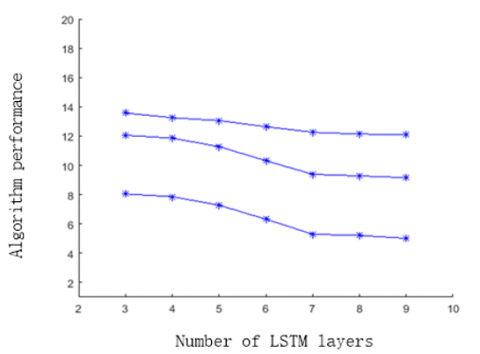Figure 7. Relationship between the number of LSTM layers and algorithm performance

3.6 Relationship between predicted value and measured value

600 samples were selected from the global and local predicted value and measured value datasets to plot the distribution maps of the predicted values and measured values of PM2.5 concentration in global and local regions (as shown in Figure 8).

The experimental results showed that, between the predicted values and the measured values, there was a fit that approximated to y=x+ε, wherein the ε was any small positive number, the distribution areas were relatively concentrated, indicating that the predicted results and the measured results were come from the same dataset.

## 8.pngFigure 8. Distribution maps of predicted values and measured values of PM2.5 concentration in global and local regions

3.7 Comparative study of algorithm prediction performance

On the same training and test dataset, under the conditions of different input parameters and different network structures, the performances of the proposed ST-LSTM algorithm, the LSTM algorithm , LSTME algorithm , STDL algorithm , DL-LSTM algorithm , SVR  and ARMA  were compared. The LSTM, LSTM E, DL-LSTM, and STDL algorithms adopted the same inputs as the TSM-LSTM algorithm, but the network structures were different.

The experimental results (Table 4) showed that: on the same training and test dataset, under the conditions of different input parameters and different network structures, the artificial neural network algorithms had the characteristics of nonlinear learning and fitting, therefore, the prediction ability of the neural network algorithms was better than that of the non-neural network algorithms. With the increase of the number of layers, the deep neural networks’ learning and abstraction capabilities were enhanced, and the prediction ability of the network algorithms was better than that of the shallow neural network algorithms. The multilayer TSM-LSTM algorithm proposed in this paper outperformed other LSTM networks in terms of the prediction performance. In summary, compared with other time series analysis algorithms, the TSM-LSTM proposed in this paper had better prediction ability.

Table 4. Comparison of algorithm performance

 Algorithm Index LSTM STDL DL-LSTM ARMA SVR LSTME TSM-LSTM RMSE 16.24 11.51 15.12 25.41 24.14 15.45 12.56 MAE 8.31 4.26 12.31 15.35 12.21 7.35 5.30 MAPE 17.54 8.15 28.14 26.32 26.78 10.69 9.39
4. Conclusions

Targeting at the problems that the current air pollutant particle concentration prediction algorithms failed to effectively utilize the feature of the long-term time dependence of particle concentration and ignored the spatial correlation feature of particle concentration, this paper proposed a PM2.5 concentration prediction algorithm (TSM-LSTM) based on spatial-temporal correlation and LSTM extension; by effectively integrating the spatial correlation and long-term time dependence of air pollutant PM2.5 concentration, the LSTM algorithm was improved and applied to the PM2.5 concentration evolution process simulation and numerical prediction. On the global and local datasets, different network structures and experimental parameters were selected to compare multiple classic algorithms, and the proposed algorithm exhibited excellent performance in prediction and simulation. The study discovered the following rules:

(1) In terms of evolution simulation and numerical prediction of air particle concentration, the deep neural networks were superior to shallow neural networks, and the shallow neural networks were superior to non-neural networks.

(2) In terms of evolution simulation and numerical prediction of air particle concentration, LSTM neural networks can better learn the long-term time dependence feature of air concentration, therefore, their simulation results and prediction performance were better than those of similar shallow neural networks.

(3) In terms of evolution simulation and numerical prediction of air particle concentration, the multi-layer LSTM neural network with temporal and spatial feature learning capabilities outperformed the traditional time series algorithms and the neural network algorithms.

(4) The model in this paper had good performance in the prediction and simulation of air quality in global and local regions.

Acknowledgment

This work is supported by the Social Science Fund of Hebei Province of China (Grant numbers: HB18TJ004).

References

 Krewski, D., Jerrett, M., Burnett, R.T., Ma, R., Hughes, E., Shi, Y., Turner, M.C., Pope, C.A., Thurston, G., Calle, E.E., Thun, M.J., Beckerman, B., DeLuca, P., Finkelstein, N., Ito, K., Moore, D.K., Newbold, K.B., Ramsay, T., Ross, Z., Shin, H., Tempalski, B. (2009). Extended follow-up and spatial analysis of the American Cancer Society study linking particulate air pollution and mortality. Research report (Health Effects Institute), (140): 5-114; discussion 115-36.

 Zheng, Y., Liu, F., Hsieh, H.P. (2013). U-Air: When urban air quality inference meets big data. In Proceedings of the 19th ACM SIGKDD International Conference on Knowledge Discovery and Data Mining, pp. 1436-1444. https://doi.org/10.1145/2487575.2488188

 Di, Q., Dai, L., Wang, Y., Zanobetti, A., Choirat, C., Schwartz, J.D., Dominici, F. (2017). Association of short-term exposure to air pollution with mortality in older adults. JAMA, 318(24): 2446-2456. https://doi.org/10.1001/jama.2017.17923

 Huang, L., Zhang, C., Bi, J. (2017). Development of land use regression models for PM2.5, SO2, NO2 and O3 in Nanjing, China. Environmental Research, 158: 542-552. https://doi.org/10.1016/j.envres.2017.07.010

 Jeong, J.I., Park, R.J., Woo, J.H., Han, Y.J., Yi, S.M. (2011). Source contributions to carbonaceous aerosol concentrations in Korea. Atmospheric Environment, 45(5): 1116-1125. https://doi.org/10.1016/j.atmosenv.2010.11.031

 Chen, J., Lu, J., Avise, J.C., DaMassa, J.A., Kleeman, M.J., Kaduwela, A.P. (2014). Seasonal modeling of PM2.5 in California’s San Joaquin Valley. Atmospheric Environment, 92: 182-190. https://doi.org/10.1016/j.atmosenv.2014.04.030

 Wang, Z., Maeda, T., Hayashi, M., Hsiao, L.-F., Liu, K.Y. (2001). A nested air quality prediction modeling system for urban and regional scales: Application for high-ozone episode in Taiwan. Water, Air, and Soil Pollution, 130: 391-396. https://doi.org/10.1023/A:1013833217916

 Saide, P.E., Carmichael, G.R., Scott, N., Gallardo, L., Osses, A.E., Mena-Carrasco, M.A., Pagowski, M. (2011). Forecasting urban PM10 and PM2.5 pollution episodes in very stable nocturnal conditions and complex terrain using WRF-Chem CO tracer model. Atmospheric Environment, 45(16): 2769-2780. https://doi.org/10.1016/j.atmosenv.2011.02.001

 Vautard, R., Builtjes, P.H.J., Thunis, P., Cuvelier, C., Bedogni, M., Bessagnet, B., Honoré, C., Moussiopoulos, N., Pirovano, G., Schaap, M., Stern, R., Tarrason, L., Wind, P. (2007). Evaluation and intercomparison of Ozone and PM10 simulations by several chemistry transport models over four European cities within the CityDelta project. Atmospheric Environment, 41(1): 173-188. https://doi.org/10.1016/j.atmosenv.2006.07.039

 Stern, R., Builtjes, P., Schaap, M., Timmermans, R., Vautard, R., Hodzic, A., Wolke, R. (2008). A model inter-comparison study focussing on episodes with elevated PM10 concentrations. Atmospheric Environment, 42(19): 4567-4588. https://doi.org/10.1016/j.atmosenv.2008.01.068

 Ge, B., Gm, J. (1976). Time series analysis: forecasting and control rev. ed. Oakland, California, Holden-Day, 31(4): 238-242.

 Li, C., Hsu, N.C., Tsay, S.C. (2011). A study on the potential applications of satellite data in air quality monitoring and forecasting. Atmospheric Environment, 45(22): 3663-3675. https://doi.org/10.1016/j.atmosenv.2011.04.032

 García Nieto, P.J., Combarro, E.F., del Coz Díaz, J.J., Montañés, E. (2013). A SVM-based regression model to study the air quality at local scale in Oviedo urban area (Northern Spain): A case study. Applied Mathematics and Computation, 219(17): 8923-8937. https://doi.org/10.1016/j.amc.2013.03.018

 Hooyberghs, J., Mensink, C., Dumont, G., Fierens, F., Brasseur, O. (2005). A neural network forecast for daily average PM10 concentrations in Belgium. Atmospheric Environment, 39(18): 3279-3289. https://doi.org/10.1016/j.atmosenv.2005.01.050

 Chen, Y., Shi, R., Shu, S., Gao, W. (2013). Ensemble and enhanced PM10 concentration forecast model based on stepwise regression and wavelet analysis. Atmospheric Environment, 74: 346-359. https://doi.org/10.1016/j.atmosenv.2013.04.002

 Yoon, H., Jun, S.C., Hyun, Y., Bae, G.O., Lee, K.K. (2011). A comparative study of artificial neural networks and support vector machines for predicting groundwater levels in a coastal aquifer. Journal of Hydrology, 396(1): 128-138. https://doi.org/10.1016/j.jhydrol.2010.11.002

 Kolehmainen, M., Martikainen, H., Ruuskanen, J. (2001). Neural networks and periodic components used in air quality forecasting. Atmospheric Environment, 35(5): 815-825. https://doi.org/10.1016/S1352-2310(00)00385-X

 Lu, W.Z., Wang, W.J., Fan, H.Y., Leung, A.Y.T., Xu, Z.B., Lo, S.M., Wong, J.C.K. (2002). Prediction of pollutant levels in causeway bay area of Hong Kong using an improved neural network model. Journal of Environmental Engineering, 128(12): 1146-1157. https://doi.org/10.1061/(ASCE)0733-9372(2002)128:12(1146)

 Mishra, D., Goyal, P. (2017). Neuro-fuzzy approach to forecast NO2 pollutants addressed to air quality dispersion model over Delhi, India. Aerosol and Air Quality Research, 16(1): 166-174. https://doi.org/10.4209/aaqr.2015.04.0249

 Antanasijevi, D.Z., Pocajt, V.V., Povrenovi, D.S., Risti, M., Peri-Gruji, A.A. (2012). PM 10 emission forecasting using artificial neural networks and genetic algorithm input variable optimization. Sci. Total Environ. 443: 511-519. https://doi.org/10.1016/j.scitotenv.2012.10.110

 Feng, Y., Zhang, W., Sun, D., Zhang, L. (2011). Ozone concentration forecast method based on genetic algorithm optimized back propagation neural networks and support vector machine data classification. Atmospheric Environment, 45(11): 1979-1985. https://doi.org/10.1016/j.atmosenv.2011.01.022

 Ma, X., Tao, Z., Wang, Y., Yu, H., Wang, Y. (2015). Long short-term memory neural network for traffic speed prediction using remote microwave sensor data. Transportation Research Part C-Emerging Technologies, 54: 187-197. https://doi.org/10.1016/j.trc.2015.03.014

 Ong, B.T., Sugiura, K., Zettsu, K. (2016). Dynamically pre-trained deep recurrent neural networks using environmental monitoring data for predicting PM2.5. Neural Computing and Applications, 27(6): 1553-1566. https://doi.org/10.1007/s00521-015-1955-3

 Prakash, A., Kumar, U., Kumar, K., Jain, V.K. (2011). A wavelet-based neural network model to predict ambient air pollutants’ concentration. Environmental Modeling & Assessment, 16(5): 503-517. https://doi.org/10.1007/s10666-011-9270-6

 Hochreiter, S., Schmidhuber, J. (1997). Long short-term memory. Neural Computation, 9(8): 1735-1780. https://doi.org/10.1162/neco.1997.9.8.1735

 Bai, Y., Zeng, B., Li, C., Zhang, J. (2019). An ensemble long short-term memory neural network for hourly PM2.5 concentration forecasting. Chemosphere, 222: 286-294. https://doi.org/10.1016/j.chemosphere.2019.01.121

 Qin, D., Yu, J., Zou, G., Yong, R., Zhao, Q., Zhang, B. (2019). A novel combined prediction scheme based on CNN and LSTM for urban PM 2.5 concentration. IEEE Access, 7: 20050-20059. https://doi.org/10.1109/access.2019.2897028

 Zhao, J., Deng, F., Cai, Y., Chen, J. (2019). Long short-term memory - Fully connected (LSTM-FC) neural network for PM2.5 concentration prediction. Chemosphere, 220: 486-492. https://doi.org/10.1016/j.chemosphere.2018.12.128

 Qi, Y., Li, Q., Karimian, H., Liu, D. (2019). A hybrid model for spatiotemporal forecasting of PM2.5 based on graph convolutional neural network and long short-term memory. Science of The Total Environment, 664: 1-10. https://doi.org/10.1016/j.scitotenv.2019.01.333

 Wen, C., Liu, S., Yao, X., Peng, L., Li, X., Hu, Y., Chi, T. (2019). A novel spatiotemporal convolutional long short-term neural network for air pollution prediction. Science of The Total Environment, 654: 1091-1099. https://doi.org/10.1016/j.scitotenv.2018.11.086

 Freeman, B.S., Taylor, G., Gharabaghi, B. (2018). Forecasting air quality time series using deep learning. Journal of The Air & Waste Management Association, 68(8): 866-886. https://doi.org/10.1080/10962247.2018.1459956

 Zhou, Y., Chang, F.J., Chang, L.C., Kao, I.F., Wang, Y.S. (2019). Explore a deep learning multi-output neural network for regional multi-step-ahead air quality forecasts. Journal of Cleaner Production, 209: 134-145. https://doi.org/10.1016/j.jclepro.2018.10.243

 Huang, C.J., Kuo, P.H. (2018). A deep CNN-LSTM model for particulate matter (PM2.5) Forecasting in smart cities. Sensors, 18(7): 2220. https://doi.org/10.3390/s18072220

 Feng, X., Li, Q., Zhu, Y., Hou, J., Jin, L., & Wang, J. (2015). Artificial neural networks forecasting of PM2.5 pollution using air mass trajectory based geographic model and wavelet transformation. Atmospheric Environment, 107: 118-128. https://doi.org/10.1016/j.atmosenv.2015.02.030

 Qi, Y., Li, Q., Karimian, H., Liu, D. (2019). A hybrid model for spatiotemporal forecasting of PM2.5 based on graph convolutional neural network and long short-term memory. Science of The Total Environment, 664: 1-10. https://doi.org/10.1016/j.scitotenv.2019.01.333

 Li, X., Peng, L., Yao, X., Cui, S., Hu, Y., You, C., Chi, T. (2017). Long short-term memory neural network for air pollutant concentration predictions: Method development and evaluation. Environmental Pollution, 231(231): 997-1004. https://doi.org/10.1016/j.envpol.2017.08.114

 Li, X., Peng, L., Hu, Y., Shao, J., Chi, T. (2016). Deep learning architecture for air quality predictions. Environmental Science and Pollution Research, 23(22): 22408-22417. https://doi.org/10.1007/s11356-016-7812-9